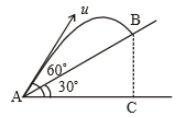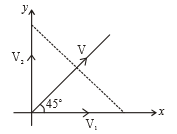A particle is projected at an angle $\theta$ with horizontal with an initital speed u. When it makes an angle $\alpha$ with horizontal, its speed v is-

1. $u\mathrm{cos}\theta$

2.

3. $\frac{u\mathrm{sin}\theta }{\mathrm{sin}\alpha }$

4. $\frac{u\mathrm{cos}\theta }{\mathrm{cos}\alpha }$

Concept Questions :-

Projectile motion
High Yielding Test Series + Question Bank - NEET 2020

Difficulty Level:

A body is projected with velocity $20\sqrt{3}$ m/s with an angle of projection 60$°$ with horizontal. Calculate velocity on that point where body makes an angle 30$°$ with the horizontal.

(1) 20 m/s

(2)

(3)

(4) 10 m/s

Concept Questions :-

Projectile motion
High Yielding Test Series + Question Bank - NEET 2020

Difficulty Level:

A particle is moving with veocity $\stackrel{⇀}{\mathrm{V}}=k\left(y\stackrel{^}{i}+x\stackrel{^}{j}\right)$; where k is constant. The general equation for path is:

1. $\mathrm{y}={\mathrm{x}}^{2}+\mathrm{constant}$

2. ${\mathrm{y}}^{2}={\mathrm{x}}^{2}+\mathrm{constant}$

3. $\mathrm{y}=\mathrm{x}+\mathrm{constant}$

4. xy=constant

Concept Questions :-

Speed and velocity
High Yielding Test Series + Question Bank - NEET 2020

Difficulty Level:

A body thrown vertically so as to reach its maximum height in t second. The total time from the time of projection to reach a point at half of its maximum height while returning (in second) is:

(1) $\sqrt{2}t$

(2) $\left(1+\frac{1}{\sqrt{2}}\right)t$

(3) $\frac{3t}{2}$

(4) $\frac{t}{\sqrt{2}}$

Concept Questions :-

Projectile motion
High Yielding Test Series + Question Bank - NEET 2020

Difficulty Level:

A particle is projected with a velocity u making an angle $\mathrm{\theta }$ with the horizontal. At any instant, its velocity v is at right angles to its initial velocity u; then v is:

1.  u cos $\mathrm{\theta }$

2.  u tan $\mathrm{\theta }$

3.  u cot $\mathrm{\theta }$

4.  u sec $\mathrm{\theta }$

Concept Questions :-

Projectile motion
High Yielding Test Series + Question Bank - NEET 2020

Difficulty Level:

A projectile is given an initial velocity of $\stackrel{^}{i}+2\stackrel{^}{j}$. The cartesian equation of its path is (g = 10 ${\mathrm{ms}}^{-2}$

1. $\mathrm{y}=2\mathrm{x}-5{\mathrm{x}}^{2}$

2. $\mathrm{y}=\mathrm{x}-5{\mathrm{x}}^{2}$

3. $4\mathrm{y}=2\mathrm{x}-5{\mathrm{x}}^{2}$

4. $\mathrm{y}=2\mathrm{x}-25{\mathrm{x}}^{2}$

Concept Questions :-

Projectile motion
High Yielding Test Series + Question Bank - NEET 2020

Difficulty Level:

A ship A is moving westwards with a speed of 10 km ${\mathrm{h}}^{-1}$ and a ship B, 100 km south of A is moving northwards with a speed of 10 km ${\mathrm{h}}^{-1}$. The time after which the distance between them becomes the shortest, is:

1. 5 hr

2. $5\sqrt{2}$ hr

3. $10\sqrt{2}$ hr

4. 0 hr

Concept Questions :-

Relative motion
High Yielding Test Series + Question Bank - NEET 2020

Difficulty Level:

Time taken by the projectile to reach from A to B is t. Then the distance AB is equal to :1. $\frac{ut}{\sqrt{3}}$

2. $\frac{\sqrt{3}ut}{2}$

3. $\sqrt{3}ut$

4. 2ut

Concept Questions :-

Projectile motion
High Yielding Test Series + Question Bank - NEET 2020

Difficulty Level:

A particle projected with kinetic energy ${\mathrm{k}}_{0}$ with an angle of projection $\mathrm{\theta }$. Then the variation of kinetic K with vertical displacement y is

1.  linear

2.  parabolic

3.  hyperbolic

4.  periodic

Concept Questions :-

Projectile motion
High Yielding Test Series + Question Bank - NEET 2020

Difficulty Level:

Three particles moving with constant velocities  and V respectively as given in the figure. After some time all three particles are in the same line, then relation among  and V is1.

2.

3.

4.

Concept Questions :-

Speed and velocity